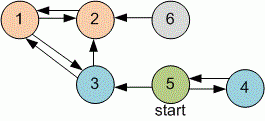favorite We need a little bit of your help to keep things running, click on this banner to learn more
Competitions

# The shortest distance

The directed graph is given. Find the shortest distance from the vertex x to other vertices of the graph.

#### Input

The first line contains two integers n and x (1n1000, 1xn) - the number of vertices in a graph and the starting vertex. Next n lines contains n numbers - the adjacency matrix of the graph: the i-th line and j-th column contains "1", if the vertices i and j are connected with the edge, and "0", if there is no edge between them. The main diagonal contains zeroes.

#### Output

Print the numbers `d1`, `d2`, ..., `dn`, separated with a space, where `di` is -1 if there is no path from x to i, or the minimal distance from x to i otherwise.Time limit 2 second
Memory limit 128 MiB
Input example #1
```6 5
0 1 1 0 0 0
1 0 0 0 0 0
1 1 0 0 0 0
0 0 0 0 1 0
0 0 1 1 0 0
0 1 0 0 0 0
```
Output example #1
```2 2 1 1 0 -1
```